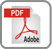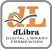# USING POLYNOMIALS OF VARIABLE DEGREES FOR SOLVING THE RELATIVE N-KODY PROBLEM

Marciniak A.

Poznań University of Technology, Institute of Computing Science
Piotrowo 3a, 60-965 Poznań, Poland
and
Adam Mickiewicz University, Faculty of Mathematics and Computer Science
Matejki 48/49, 60-769 Poznań, Poland

R e c e i v e d 13 June, 2 0 0 1

### Abstract:

A variable order method for solving the planetary type N-body problem, which is based on an
approximation by polynomials of variable degrees, is proposed. We present an algorithm for finding such polynomials, notes on the stability and convergence of the method, and some selected numerical examples.

### References:

 Bordovicyna.T. V., Modern Numerical Methods in Celestial Mechanics Problems (in Russian), Izdatel’stvo ‘Nauka’, Moscow 1984.
 Brouwer, D., Clemence, G. M., Methods of Celestial Mechanics, Academic Press, New York 1961.
 Bulirsch, R., Stoer, J., Numerical Treatment of Ordinary Differential Equations by Extrapolation Methods, Numer. Math. 8 (1966), 1-13.
 Everhart, E., Implicit Single Sequence Methods for Integrating Orbits, Celestial Mech. 10 (1974), 35-55.
 Gragg, W. B., On Extrapolation Algorithms of Ordinary Initial Value Problems, J. SIAM Numer Anal. 2 (1965), 384-403.
 Greenspan, D., Discrete Newtonian Gravitation and the N-body Problem, Utilitas Math. 2(1972), 105-126.
 Greenspan, D., Conservative Numerical Methods for
 Marciniak, A., Energy Conserving, Arbitrary Order Numerical Solutions of the N-body Problem, Mumer. Math. 45 (1984), 207-218.
 Marciniak, A., Numerical Solutions of the N-body Problem, D. Reidel Publishing Co., Dordrecht 1985.
 Marciniak, A., Arbitrary Order Numerical Solutions Conserving the Jacobi Constant in the Motion Nearby the Equilibrium Points, Celestial Mech. 40 (1987), 95-110.
 Marciniak, A., Selected Numerical Methods for Solving the N-body Problem (in Polish), Thesis No. 213, Poznań Univ. of Technology, Poznań 1989.
 Marciniak, A., Arbitrary Order Numerical Methods Conserving Integrals for Solving Dynamic Equations, Computers Math. Applic. 28 (1994), 33-43.
 Nacozy, P. E., The Use of Integrals in Numerical Integration of N-body Problem, Astrophys. Space Sci. 14 (1971), 40-41.
 Pollard, H., Mathematical Introduction to Celestial Mechanics, Prentice Hall, New Jersey 1966.
 Steffensen, K. F., On the Problem of Three Bodies in the Plane, Kong. Danske Videnskab. Seskob., Mat. Fys. Medd. 13 (1957).
 Stetter.H. L, Analysis of DiscretizationMethodsforOrdinaiy Differential Equations, Spńngev-Veńag, Berlin 1973.
 Wierzbiński, S., Celestial Mechanics (in Polish), PWN, Warsaw 1973.